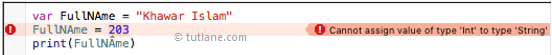# Swift Data Types

Generally, in any programming language we use data types to store and manipulate the data in variables based on the type of data like integers, strings, characters, bool, etc.

Whenever we create the variable in swift programming language, automatically the required space is reserved in memory for that variable and the space allocation in memory is varied based on the type of variables like integer or float or double, etc.

## Swift Basic Data Types

The following are the basic and most frequently used data types available in the swift programming language.

Data TypeDescriptionExample
Integer Int or UInt stand for Integer and used for numbers like 1, 2, 3, 4, 5. We can use Int32, Int64 to define 32 or 64-bit signed integer, whereas UInt32 or UInt64 to define 32 or 64-bit unsigned integer variables. For example, 345566 and -345566.

100

Float Float stands for floating value and it is used to represent numbers with fraction values like 245.344 and etc. It is used to hold the numbers with larger or smaller decimal points like 3.14, and -455.3344.

3.14

-455.3344

Double Double stands for Double means if we want to allocate the large number with fraction value then we use Double as a float data-type. We can use it as a 64-bit floating-point number and Double is more precise than Float. For example, 345.344544, and -4554.3455543.

345.344544

-4554.3455543

Bool Bool represents only two values either TRUE or FALSE. We can use Bool to check whether certain conditions met or not.

let i = 10

if i> 20 {

}

String String is a combination of Characters. We can define a string data type by adding double quotes to our text like “Hello World”.

""

"welcome to tutlane"

Character It represents a single alphabet character like “H”, “e”, “L”, “L”, “o”. “H”
Optional It represents a variable can hold value or no value.

## Swift Data Type Ranges

The following table shows the details like how much memory space occupied by a variable based on the data type and minimum & maximum values range for each data type.

Data TypeMemory AllocationMin & Max Range
Int8 1byte -127 to 127
UInt8 1byte 0 to 255
Int32 4bytes -2147483648 to 2147483647
UInt32 4bytes 0 to 4294967295
Int64 8bytes -9223372036854775808 to 9223372036854775807
UInt64 8bytes 0 to 18446744073709551615
Float 4bytes 1.2E-38 to 3.4E+38 (~6 digits)
Double 8bytes 2.3E-308 to 1.7E+308 (~15 digits)

## Type Aliases

In swift, by using typealiases keyword we can create a new name for an existing type. The typealiases are useful whenever we want to create a meaningful name for our existing types.

Following is the syntax of creating an alternative name to the existing type using typealias keyword.

typealias <Name> = <type>

Following is the simple example of using typealias to create an alternative name for the “String” type.

typealias PersonName = String

var Name : PersonName =  "Tutlane"

print(Name)

If you observe the above example we created an alternative name “PersonName” to the “String” data type.

When we compile the above the code, the compiler gives the following output

Tutlane

## Type Safety

Swift is a type-safe programming language that means once we define a variable with one data type then it’s not possible to assign another data type value to it.

Suppose if we define a variable with String data type and if we try to pass an integer value then the compiler will throw an error like as shown following because swift is a type-safe language and it performs type checks while compiling our code and flags any mismatched types as errors.## Type Inference

In swift Type inference enables a compiler to deduce the type of a particular expression automatically when it compiles your code, simply by examining the values you provide.

Following is the simple example to deduce the variable value, when the compiler found that it adds the floating values to an integer.

var varA = 53453

print(varA)

var varB = 53453.453453

print(varB)

var varC = 53 + 0.45353

print(varC)

When we run above program we will get output like as shown below.

53453

53453.453453

53.45353